## Alphabet Tricks, Examples and Online Test

Problems under this segment are the very important part of the questions asked in various competitive exams to be conducted for the purpose of requirement of officers and clerks. Particularly for getting job in banking sector, this type of questions can not be ignored.

While solving the problems based on alphabet, you must have in your mind the exact positions of every letters of alphabet in forward order as well as in backward or reverse order

### Types of Problems

1. General series of alphabet
2. Words in alphabetical order
3. Problems of word formation
4. Problems of letter gap

Now we will discuss all the above types of problems one by one in detail.

### 1. General Series of Alphabet

Example 1. Which of the following options is seventh to the right of the 13th letter from the left in a forward Alphabet series?

1. R
2. T
3. V
4. W

Solution: Now the question is how to solve it?

1st of all we will write the forward alphabet series as given below: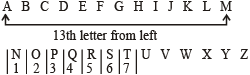From above series it is clear that M is the 13th letter from left and to the right of M (13th letter from left), T is the 7th letter.

Hence (2) is the correct option.

Here, we have solved this problem with a general method. But this type of problem can also be approached through quicker method that will help you save some extra consumed time.

#### Rules for quicker method

• (a) If both the directions are same then subtraction of numbers takes place.
• (b) If the directions are opposite then addition of numbers takes place.

Now, for solving the sample question we apply this rule. As we want to find out the 7th letter to the right of the 13th letter from the left, the directions are opposite and thus rule (b) will be applied here.

Hence we add 7 + 13 = 20.
Therefore, the answer will be 20th from left.
Also, 20th from left mean 26 – 20 + 1 = 7th from right.
∴ 20th letter from left = T
Also 7th letter from right = T

This method also gives the answer choice (2).

After solving the sample question, you must have noticed that the above mentioned trick is to calculate the actual position of the required letter before going to search for it.

Other variations of such type of problems

Example 2. If alphabet series is given in backward or reverse order, then find out the eighth letter to right of O?

1. H
2. G
3. U
4. X

Solution: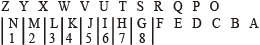It’s clear (2) is the correct answer.

Note: Even with the forward alphabet series we can solve this problem because the letter which is eight to the right of O in the reverse order alphabet series must be eight to the left of O in forward alphabet series.

Example 3. If the 1st half of the alphabet is written in reverse order, then find out the letter that would be 20th letter from the right.

1. G
2. F
3. D
4. H

Solution: As the 2nd half is not reversed, the 1st 13 letter would be same when we do counting from right. But not letters coming after 13th will be actually from the left.

Hence 14th letter from right would be A;

15th would be B;

16th would be C

and we move further in the same manner. Hence from left which is G.

∴ Option (1) is the correct answer.

#### Important Tips

While solving the problems based on alphabet, you must have in your mind the exact positions of every letters of alphabet in forward order as well as in backward or reverse order as given below:

Letters positions in forward alphabetical order:

 A B C D E F G H I J K L M 1 2 3 4 5 6 7 8 9 10 11 12 13 N O P Q R S T U V W X Y Z 14 15 16 17 18 19 20 21 22 23 24 25 26

Letters positions in backward or reverse alphabetical order:

 A B C D E F G H I J K L M 26 25 24 23 22 21 20 19 18 17 16 15 14 N O P Q R S T U V W X Y Z 13 12 11 10 9 8 7 6 5 4 3 2 1

mth element to be counted from left to right of a series of x characters is equal to (x + 1 – m)th element to be counted from right to left of that series. This rule can be better illustrated by an example which is given below:
Let us take the forward order alphabet series,

As we know that English alphabet has 26 characters, hence, we have x = 26.
Now suppose, we have to find out the position of K in the above given series counting from right to left.
Position of ‘K’ in the English alphabet from left to right is 11. Thus m = 11

∴ Position of K in the above given series from right to left would be (26 + 1 – 11) = 16

How to solve problems when letters are dropped or deleted at regular intervals?

Sample Question: If every 3rd letter from left to right of English alphabet is deleted, then what would be the 6th letter from left in the new series obtained?

Solution: General method: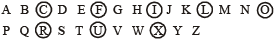Here, deleted letters have been encircled and we find the new series as given below: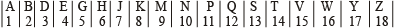It is clear, that 6th letter from left in the new series is H.

#### How to solve problems based on the backward (reversed) alphabet series?

While solving problems based on general series of alphabet, we come across the various cases. In some cases we see that whole alphabet series is reversed but in some other cases 1st half of the series is reversed, or second half of the series is reversed or many segments of the alphabet series are reversed.

Let us take a case when a forward order alphabet series get reversed in three segments. In 1st segment 8 letters get reversed; in 2nd segment the next 8 letters get reversed and in the 3rd segment the remaining 10 letters get reversed. Just see the presentation given below: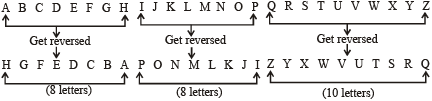Now if you are asked to find out the 4th letter from left in the new obtained series, then through general method, we simply do counting from left in the new series and find out our required answer as ‘E’ because ‘E’ is at 4th position from left in the new obtained series. But while solving such type of problems, we have to do some time consuming formalities like

1. writing the original series
2. writing and reversing the letters of original series as per the question says and
3. counting them to get the required answer.

Such time consuming processes can be avoided if we go through quicker approach.

#### Quicker approach

It is clear that 4th letter from left in the new obtained series falls into first segment which has 8 letters. Hence 4th letter in the new obtained series = (8 + 1 – 4) = 5th letter from the left in the original series. As we know that exact position of 5th letter from left in the original alphabet series is the position of E. Hence E is our required answer.

If we have to find out 18th letter from left in the new obtained series, then that will be 16 + (10 + 1 – 2) = 25th letter from left in the original alphabet series (why?) which is Y.

In fact, while finding out 18th letter, we can easily see that 18th letter is the 2nd letter of 3rd segment and hence it will be not affected by 1st two segments having 8 letters each. In other words to find out 18th letter in the new obtained series, we have to find out the 2nd letter in the 3rd segment. This is the reason we find out the 2nd letter in the 3rd segment and then add the 16 letters of 1st two segment to get the 18th letter in the new obtained series. From this, we find that 18th letter from left in the new obtained series is the 25th letter from left in the original series. As 25th letter from left in the original series is Y. It (Y) will be our required answer.

Edudose users are advised to practice such type of problems as much as possible and after a certain time type will notice that they have get a skill to solve such problems in a few seconds and that too, without the use of pen and paper.

#### How to solve if positions of letters are interchanged?

There is no any rule for such type of problems. Only the hard practice can given you a skill to solve such questions in a quick time.

Example 5. If A and C interchange their places, B and D interchange their places, F and H interchange their places and so on, then which letter will be 5th to the left of Q?

1. P
2. N
3. M
4. T

Solution: As per the question the interchanges take place as follows:Here we can see that Q interchanges with S. Then to left of Q, the 5th letter would be P because P interchanges with N.

How to find the middle letter?

Case I: Remember that if mth and nth letter from the left in the English alphabet are given then

Middle letter =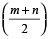th letter from the left.

Example 6. Which letter will be midway between 8th letter from the left and 16th letter from the left in the English alphabet?

Solution: Here m = 8 and n = 16

then middle letter =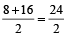= 12th letter from left in the alphabet = L

Case II: Remember that if mth and nth letter from the right in the English alphabet are given then Middle letter =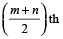letter from right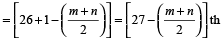letter from the left in the English alphabet.

Example 7. Which letter will be midway between 8th letter from the right and 16th letter from the right in the English alphabet.

Solution: Middle letter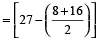th letter from left in the alphabet.

or middle letter = (27 – 12) = 15th letter from left = 0

Note: In case I and case II (m + n) must be divisible by 2.

Case III: Remember that if the mth letter from the left and the nth letter from the right are given then middle letter =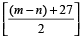th letter from the left in the alphabet.

Example 8. Which letter will be midway between 8th letter from the left and 15th letter from the right?

Solution: Here m = 8 and n = 15.

Then middle letter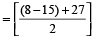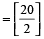= 10th letter from left in the English alphabet = J.

Note: In case III (m – n) + 27 must be divisible by 2.

### 2. Words in alphabetical order

In such type of questions, words are given and you have to find out which word will appear in the dictionary 1st or 2nd or 3rd or 4th etc.

Example 9. Which of the following words will come 2nd in the dictionary?

1. Name
2. Shame
3. Fame
4. Came

Solution:

‘Came’ comes 1st in the dictionary.
‘Fame’ comes 2nd in the dictionary
‘Name’ comes 3rd in the dictionary
‘Shame’ comes 4th in the dictionary

∴ (c) is the required answer.

Example 10. Find out the word coming last in the dictionary.

1. Large
2. Long
3. Lust
4. Love

Solution:

• Step I: In this question the 1st letter of all the words are same. Hence, from 1st letter we can not find out this arrangement in the dictionary.
• Step II: We move on the 2nd letter of the words and find that 2nd letter of Large is ‘a’; 2nd letter of Long is ‘o’; 2nd letter of Lust is ‘u’ 2nd letter of Love is ‘O’. Now its clear that in the dictionary ‘a’ comes before ‘o’ & u. Hence the word ‘Large’ comes 1st in the dictionary.
• Step III: Now we will compare the remaining three words ‘Long’, Lust and ‘Love’. Here, when we see the 2nd letter of these words we find ‘O’ comes before ‘U’ in the dictionary. Hence we can come to the conclusion that the words ‘Long’ and ‘Love’ will definitely not the last word. Thus we came to our required answer that the word ‘Lust’ or option (c) will definitely come last in the dictionary.

∴ Option (3) will be our answer. But if we want to know the 2nd and 3rd word also then we can move on to the next step.

• Step IV: In the words ‘Long’ and ‘Love’, 1st two letters are common. Therefore, to know the arrangement of these two words in the dictionary we move on to the third letter. Third letter in the word ‘Long’ is ‘N’ and in ‘Love’ the third letter is ‘v’. As ‘n’ comes before ‘v’ alphabetically, the word ‘Long’ will come before the word ‘Love’ in the dictionary. Hence, it is clear that ‘Long’ comes 2nd and ‘Love’ comes 3rd in the dictionary.
• Step V: Find arrangement : (1) Large (2) Long (3) Love (4) Lust.

### 3. Problems of word formation

In such problems a word is given and you have to find out the number of words to be formed out of some letters drawn from that particular word.

Example 11. How many meaningful words can be formed from the 3rd, 4th, 6th and 8th letter of the word ‘CONTROVERSIAL’?

Solution: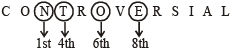Now from letters N T O and E, the two words ‘NOTE’ and ‘TONE’ can be formed.

### 4. Problems of letter gap

Case I:

Example 12. How many pairs of letters are there in the word ‘DREAMLAND’ which have as many letters between them as in the English alphabet?

Solution: Here, we are asked to solve problem according to English alphabet. In this case we have to count both ways. It does mean that we have to count from left to right and from right to left. Let us see the following presentation:The above presentation makes it clear that the required pairs of letters are 4. (Pairs: DA, EA, ML and LN)

Case II:

Example 13. How many pairs of letters are there in the word ‘DREAMLAND’ which have the same number of letters between them as in the English alphabet in the same sequence.

Solution: Here we are asked to solve problems according to the alphabetical sequence. It does mean that we have to do counting only from left to right. Let us, see the following presentation: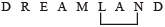The above presentation makes it clear that the required pair of letters is only 1 (Pair: LN)